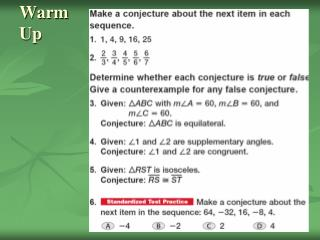Download PresentationWarm UpWarm Up - PowerPoint PPT Presentation

Download PresentationWarm Up
An Image/Link below is provided (as is) to download presentation

Download Policy: Content on the Website is provided to you AS IS for your information and personal use and may not be sold / licensed / shared on other websites without getting consent from its author. While downloading, if for some reason you are not able to download a presentation, the publisher may have deleted the file from their server.

- - - - - - - - - - - - - - - - - - - - - - - - - - - E N D - - - - - - - - - - - - - - - - - - - - - - - - - - -
Presentation Transcript

1. WarmUp

2. Warm Up Answers

3. Theorem and Proof • A theorem is a statement or conjecture that has been shown to be true. • Theorems can be used like a definition or postulate to justify other statements are true. • A proof is a logical argument in which each statement made is supported by a statement that is accepted as true. • A paragraph proof or informal proof is one type of proof.

4. 2.5 Algebraic Proof • Algebraic proofs use algebra to write two-column proofs. • Two-Column Proofs or formal proofs contains statements and reasons organized into 2 columns. • Each step is called a statement and the properties that justify each step are called the reasons.

5. Properties of Equality for Real Numbers • Reflexive Property: a = a • Symmetric Property: if a = b, then b = a • Transitive Property: if a=b, and b=c, then a=c • Addition Property: if a=b, then a+c = b+c • Subtraction Property: if a=b, then a-c = b-c • Multiplication/Division: if a=b, then ac = bc • Substitution Property: if a=b, then a may be replaced by b in any equation or expression • Distributive Property: a(b+c) = ab + ac

6. Example Complete the following proof. Given: 5- ½ x = 1 Prove: 8 = x Statements Reasons • 5 – ½ x = 1 1. Given • 5 – ½ x – 5 = 1 – 5 2. ____________ • - ½ x = -4 3. ____________ • _______________ 4. Multiplication • x = 8 5. _____________ • 8 = x 6. _____________

7. Answer Complete the following proof. Given: 5- ½ x = 1 Prove: 8 = x Statements Reasons • 5 – ½ x = 1 1. Given • 5 – ½ x – 5 = 1 – 5 2. Subtraction • - ½ x = -4 3. Substitution • -2( ½ x) = -2(-4) 4. Multiplication • x = 8 5. Substitution • 8 = x 6. Symmetric Prop.